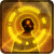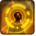# Fusion RoundConditions

Used by

## Related effects, buffs and debuffs

Please click on an effect below to view its details.

• [does not expire]Fusion Round

 Slot: Debuff Duration: passive Tick rate: does not tick # occurrences: 0 Target overrides: [target override] Aoe Sphere
• On Apply

Perform the following actions:

• Immobilize but allow rotation
• When channel ended
• [target override]

Only when the following conditions are met:

• <
• If PRIMARY TARGET is alive
AND
• If target is an enemy
>

Perform the following actions:

• Add effect #2 to TARGET from TARGET
• Play appearance epp . flashpoint . corellia . republic . enemy . boss . boss_2 . wounding_shot_fire, dependent on calling effect
• When channel finished

Perform the following actions:

• Global Cooldown
- Duration = (time) 1.5s
• [0s] [not displayed]Effect #2

 Slot: Debuff Duration: 0s Tick rate: does not tick # occurrences: 0 Conditions: Can only by called by other effects
• On Apply

Only when the following conditions are met:

• <
• If PRIMARY TARGET has an effect with the tag 4489478895498006937
AND
• If target is an enemy
>

Perform the following actions:

• Add effect #5 to TARGET from TARGET
• On Apply

Only when the following conditions are met:

• <
• If PRIMARY TARGET does not have any effect with the tag 4489478895498006937
AND
• If target is an enemy
>

Perform the following actions:

• Add effect #3 to TARGET from TARGET
• Add effect #4 to TARGET from TARGET
• [0s] [not displayed]Effect #3

 Slot: Debuff Duration: 0s Tick rate: does not tick # occurrences: 0
• [0s] [not displayed]Effect #4

 Slot: Debuff Duration: 0s Tick rate: does not tick # occurrences: 0 Conditions: Can only by called by other effects
• On Apply

Only when the following conditions are met:

• If difficulty is set to 16-man

Perform the following actions:

• Spell Damage
- Unknown (609) = (bool) false
- Damage Type = (int) 3
- Slot = (int) 1
- Spell Type = (int) 3
- Level Cap = (int) 0
- Amount Max = (float) 0
- Amount Min = (float) 0
- Amount Percent = (float) 0
- Threat Percent = (float) 0
- Standard Health Percent Max = (float) 0.18
- Standard Health Percent Min = (float) 0.18
- Amount Modifier Percent = (float) 0
- Coefficient = (float) 0
- Health Steal Percentage = (float) 0
• On Apply

Only when the following conditions are met:

• If difficulty is set to 8-man SM

Perform the following actions:

• Spell Damage
- Unknown (609) = (bool) false
- Damage Type = (int) 3
- Slot = (int) 1
- Spell Type = (int) 2
- Level Cap = (int) 0
- Amount Max = (float) 0
- Amount Min = (float) 0
- Amount Percent = (float) 0
- Threat Percent = (float) 0
- Standard Health Percent Max = (float) 0.08
- Standard Health Percent Min = (float) 0.08
- Amount Modifier Percent = (float) 0
- Coefficient = (float) 0
- Health Steal Percentage = (float) 0
• On Apply

Only when the following conditions are met:

• If difficulty is set to 8-man HM

Perform the following actions:

• Spell Damage
- Unknown (609) = (bool) false
- Damage Type = (int) 3
- Slot = (int) 1
- Spell Type = (int) 2
- Level Cap = (int) 0
- Amount Max = (float) 0
- Amount Min = (float) 0
- Amount Percent = (float) 0
- Threat Percent = (float) 0
- Standard Health Percent Max = (float) 0.2
- Standard Health Percent Min = (float) 0.2
- Amount Modifier Percent = (float) 0
- Coefficient = (float) 0
- Health Steal Percentage = (float) 0
• [0s] [not displayed]Effect #5

 Slot: Debuff Duration: 0s Tick rate: does not tick # occurrences: 0 Conditions: Can only by called by other effects
• On Apply

Only when the following conditions are met:

• If difficulty is set to 16-man

Perform the following actions:

• Spell Damage
- Unknown (609) = (bool) false
- Damage Type = (int) 4
- Slot = (int) 1
- Spell Type = (int) 3
- Level Cap = (int) 0
- Amount Max = (float) 0
- Amount Min = (float) 0
- Amount Percent = (float) 0
- Threat Percent = (float) 0
- Standard Health Percent Max = (float) 0.2
- Standard Health Percent Min = (float) 0.2
- Amount Modifier Percent = (float) 0
- Coefficient = (float) 0
- Health Steal Percentage = (float) 0
• On Apply

Only when the following conditions are met:

• If difficulty is set to 8-man SM

Perform the following actions:

• Spell Damage
- Unknown (609) = (bool) false
- Damage Type = (int) 4
- Slot = (int) 1
- Spell Type = (int) 2
- Level Cap = (int) 0
- Amount Max = (float) 0
- Amount Min = (float) 0
- Amount Percent = (float) 0
- Threat Percent = (float) 0
- Standard Health Percent Max = (float) 0.2
- Standard Health Percent Min = (float) 0.2
- Amount Modifier Percent = (float) 0
- Coefficient = (float) 0
- Health Steal Percentage = (float) 0
• On Apply

Only when the following conditions are met:

• If difficulty is set to 8-man HM

Perform the following actions:

• Spell Damage
- Unknown (609) = (bool) false
- Damage Type = (int) 4
- Slot = (int) 1
- Spell Type = (int) 3
- Level Cap = (int) 0
- Amount Max = (float) 0
- Amount Min = (float) 0
- Amount Percent = (float) 0
- Threat Percent = (float) 0
- Standard Health Percent Max = (float) 0.2
- Standard Health Percent Min = (float) 0.2
- Amount Modifier Percent = (float) 0
- Coefficient = (float) 0
- Health Steal Percentage = (float) 0